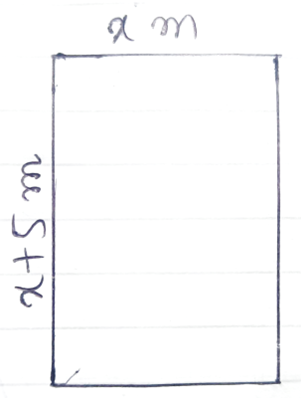The length of the garden is 5 m longer than its width and the area is 14 m2. How long is the garden?

# The length of the garden is longer than its width and the area is . How long is the garden?

1. A
2. B
3. C
4. D

Fill Out the Form for Expert Academic Guidance!l

+91

Live ClassesBooksTest SeriesSelf Learning

Verify OTP Code (required)

### Solution:

Let the width of the garden $\left(b\right)$ be .
Therefore, length of the garden $\left(l\right)$ will be .
Now, as the length and the width of the garden are not equal, therefore, the garden is in a rectangular shape as shown in the figure alongside.
Therefore, as the area of the garden is given to us, therefore, we will be using the formula of the area of the rectangle to find the length and the width of the garden.
We know, Area of the rectangular garden $=l×b$.Given: Area of the garden .
Now, put the values,
$⇒\mathit{Area}=l×b$
$⇒14=\left(x+5\right)×x$
$⇒14={x}^{2}+5x$
$⇒{x}^{2}+5x-14=0$
$⇒{x}^{2}+\left(7-2\right)x-14=0$
$⇒{x}^{2}+7x-2x-14=0$
$⇒x\left(x+7\right)-2\left(x+7\right)=0$
$⇒\left(x+7\right)\left(x-2\right)=0$
Either,
$⇒x+7=0$
$⇒x=-7$
Or,
$⇒x-2=0$
$⇒x=2$
As we know that the length and width of the garden cannot be negative, therefore,
$⇒x\ne -7$
Hence, $x=2$.
Therefore,
$⇒$Width of the garden
$⇒$ Length of the garden

## Related content

 Area of Square Area of Isosceles Triangle Pythagoras Theorem Triangle Formula Perimeter of Triangle Formula Area Formulae Volume of Cone Formula Matrices and Determinants_mathematics Critical Points Solved Examples Type of relations_mathematics+91

Live ClassesBooksTest SeriesSelf Learning

Verify OTP Code (required)